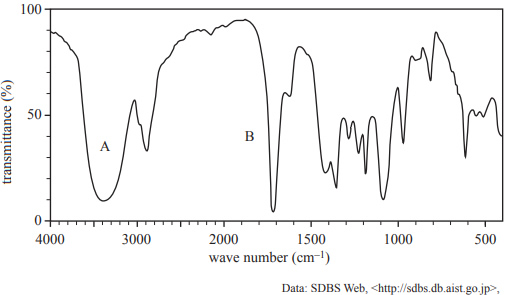Spectroscopy (2019 VCE) 1) An unknown organic compound contains carbon, hydrogen and oxygen. It is known that: • the compound does not contain carbon-to-carbon double bonds (C=C) • the molecular ion peak is found at a mass-to-charge ratio (m/z) of 74 • the 13C NMR has three distinct peaks. a. A small peak in the mass spectrum can be identified at m/z = 75. Explain the presence of this peak Solutionb) Use the information provided to give two possible molecular formulas for this compound Solutionc) The 1H NMR spectrum of the compound shows three sets of peaks with a peak area ratio of 3:2:1. What does this information tell you about the structure of the compound and its molecular formula? Justify your answer by referring to the information given about the peaks in the 1H NMR spectrum. Solutiond) There are many structural isomers of this compound. Draw the structural formulas of two possible isomers. Solutione) The infra-red (IR) spectrum of the compound is shown below.i. Identify the functional groups responsible for the absorption peaks labelled A and B in the IR spectrum. ii. Using the 1H NMR information given in part c. and the IR spectrum provided above, draw the structural formula of the compound. Solution